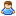1.This forum is obsolete and read-only. Feel free to contact us at support.keenswh.com

# Help my script :)

Discussion in 'Programming (In-game)' started by am1damaru, Jul 7, 2020.

This last post in this thread was made more than 31 days old.
1. ### am1damaruTrainee Engineer

Messages:
11
Hi, I’m just learning to program in the game, and I have not encountered such a level. The creak shows the fullness of the container on the screen. Containers should be named BOX_0, BOX_1, etc. The script works and I get the information on the screen, but I don’t know if I am doing it right.For example, here I led to a fractional number, because division cannot be applied to FixedPoint.

Code:
`(float)INV.CurrentVolume `
Code:
```public void Main(string argument, UpdateType updateSource)
{

//the number of containers is specified manually
int BoxCount = 3;

string str;
float volume;
float mVolume;
string error;

IMyCargoContainer BoxInv;
IMyInventory INV;

IMyTextSurface LCD_1 = GridTerminalSystem.GetBlockWithName ("LCD1") as IMyTextSurface;

str = "";
volume = 0;
mVolume = 0;
error = "";

for(int i=0; i < BoxCount; i++){
try{
BoxInv = GridTerminalSystem.GetBlockWithName ("BOX_" + i) as IMyCargoContainer;

INV = BoxInv.GetInventory(0);
str += "Инвентарь #" + i + ": " + Math.Truncate((float)INV.CurrentVolume / (float)INV.MaxVolume * 100)  + "% \n";
volume += (float)INV.CurrentVolume;
mVolume += (float)INV.MaxVolume;
}catch{
error =  "\nОшибка: Найдено " + i + " контейнера из " + BoxCount + "\n";
}
}
str += "Общая: " + Math.Truncate(volume / mVolume * 100) + "%";

LCD_1.WriteText (str + error, false);

}```
Help with advice, tips.2. ### DMOriginTrainee Engineer

Messages:
18
IMyInventory::CurrentVolume and IMyInventory::MaxVolume are of type MyFixedPoint. This type comes with some conversion operators. So, yes.. you can convert it to float or double, for example. The stored value is Liter.

Code:
```		public static implicit operator MyFixedPoint(int i);
public static explicit operator double(MyFixedPoint fp);
public static explicit operator float(MyFixedPoint fp);
public static explicit operator decimal(MyFixedPoint fp);
public static explicit operator MyFixedPoint(decimal d);
public static explicit operator MyFixedPoint(double d);
public static explicit operator MyFixedPoint(float d);
public static explicit operator int(MyFixedPoint fp);
```
For String Conversion it's better to use the formatting functionality of the ToString() function. For example:
Code:
```string str = \$"{((curValue / maxValue) * 100f).ToString("#0.00#")}%";
```
The next one is.. don't forget to check if "BoxInv" isn't "null". Or you risk that your code crash.

•Like x 1
3. ### am1damaruTrainee Engineer

Messages:
11
string str = \$"{((curValue / maxValue) * 100f).ToString("#0.00#")}%";

Highlighted what I did not understand.

4. ### MalwareMaster Engineer

Messages:
9,867
What @DMOrigin is using here is called an "interpolated string". It combines text and variables for you. However the ToString is unnecessary. It should be

Code:
```string str = \$"{((curValue / maxValue) * 100f):#0.00#}%";
```
It might be slightly easier to understand if we split the code up a bit.
Code:
```// Create the percentage value by dividing the current value with the maximum value, and multiply with a literal float of the value 100 (that's what the [B]f[/B] means: that this is a value of the float type.
float percentage = (curValue / maxValue) * 100f;

// Create a percentage string, having two decimals of precision, and ending with a percentage sign.
var str = \$"{percentage:#0.00#}%";
```
What happens is that it starts creating a string that can incorporate value formatting. That's what the \$"" means. What is inside the {} are formatted values. In this case it will read the percentage variable, then use the formatting value #0.00#, and add it to the string as a two-decimal value. Then the macro ends with the }, and the rest is just literal text - so it adds a %.

Thus; if curValue is 5 and maxValue is 10, this formatting will return "50.00%".

Another example for comparison:
Code:
`\$"Literal text {variable1:format} {variable2} more literal text";`

•Like x 1
•Agree x 1
5. ### am1damaruTrainee Engineer

Messages:
11
Oh, thanks @DMOrigin @Malware